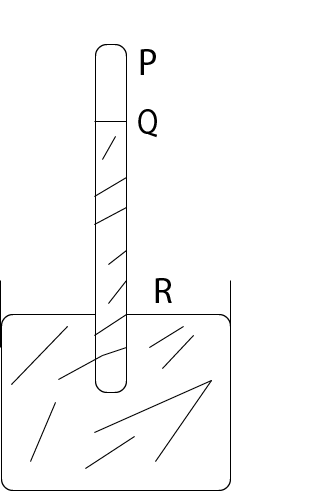## Physics 2018 Past Question | WAEC

Study the following past questions and answers for JAMBWAEC  NECO and Post-JAMB. Get prepared with official past questions and answers for upcoming examinations.

1. The S.I unit for sound energy is

• A. Hz
• B. cd
• C. dB
• D. J

2. The general definition of elastic modulus is

• A. $$\frac{stress}{strain}$$
• B. $$\frac{strain}{stress}$$
• C. $$stress \times strain$$
• D.$$\sqrt{\frac{stress}{strain}}$$

3.The diagram above illustrates a simple barometer. Which distance measures the atmospheric pressure?

• A. PQ
• B. QR
• C. RS
• D. QS

4. The property of a body to remain at rest or to continue in uniform motion in a straight line is called

• A. momentum
• B. Inertia
• C. impulse
• D. Energy

5. During a training session, two footballers pass a ball repeatedly between each other. The ‘to and fro’ motion of the ball is not simple harmonic because the

I. Acceleration of the ball is not directed towards a fixed point

II. Restoring force is not directed towards the centre

III. Acceleration of the ball is not directly proportional to the displacement from a fixed point

Which of the statements above are correct?

• A. I and III only
• B. I and II only
• C. II and III only
• D. I, II and III

6.  A dam is able to hold a large quantity of water because its wall is

• A. thickest at the top
• B. thickest at the middle
• C. thickest at the bottom
• D. of the same thickness throughout

7.

A body of mass moving around a circle of radius with a uniform speed experiences a centripetal force F. The work done by the centripetal force on it is

• A. Fv
• B. zero
• C. Fmr
• D. Fr / m

8.A block is acted upon by TWO horizontal forces as illustrated in the diagram above. The block accelerates at 1.5ms−². Calculate the mass of the block.

• A. 6kg
• B. 9kg
• C. 10kg
• D. 15kg

9. Which of the following quantities are dimensionally independent?

I. Dielectric constant    II. Momentum   III. Efficiency   IV. Refractive index

• A. I and II
• B. II, III and IV
• C. I, II, III and IV
• D. I, III and IV

10.  A body is projected with an initial velocity u at angle $$\theta$$ to the horizontal. If $$R_{max}$$ is the maximum range of the projectile, what does the relation $$\frac{u^{2}}{R_{max}}$$ represent?

• A. The vertical distance covered
• B. Acceleration due to gravity
• C. The horizontal distance covered
• D. The acceleration of the body

## All Subjects

Subscribe
Notify ofInline Feedbacks